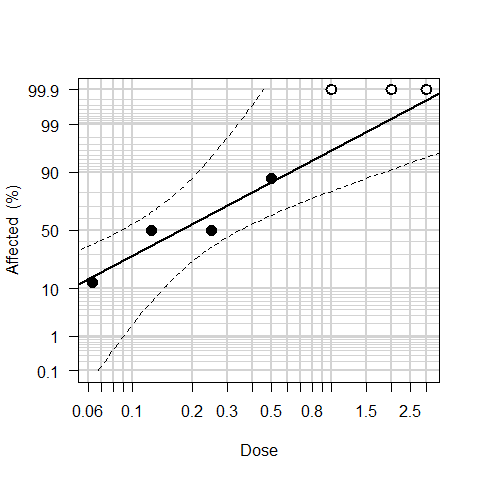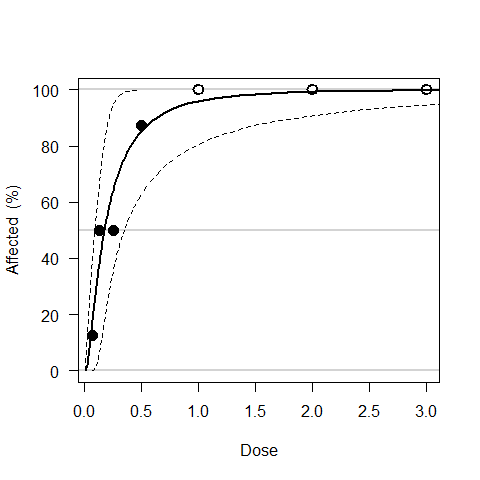Introduction to LW1949

2017-03-20

The R package LW1949 automates the steps taken in Litchfield and Wilcoxon’s (1949) manual approach to evaluating dose-effect experiments (Adams et al. 2016). Letting the computer do the work saves time and yields the best fit possible using the Litchfield Wilcoxon approach (by minimizing the chi-squared statistic). You can also try a brief demonstration of LW1949 in this web app.

Install

Install

install.packages("LW1949")

and load the LW1949 package.

library(LW1949)

Prepare data

Use the dataprep function to create a data frame with the results of a dose-effect experiment. Provide information on three key input variables,

• chemical concentration (dose),
• total number tested (ntot), and
• number affected (nfx).
conc <- c(0.0625, 0.125, 0.25, 0.5, 1, 2, 3)
numtested <- rep(8, 7)
numaffected <- c(1, 4, 4, 7, 8, 8, 8)
mydat <- dataprep(dose=conc, ntot=numtested, nfx=numaffected)

The dataprep function puts the input variables into a data frame along with several new variables,

• record number (rec),
• proportional effects (pfx),
• log10 transformed dose (log10dose),
• probit transformed effects (bitpfx),
• an effects category (fxcateg) identifying none (0), partial (50), and complete (100) effects, and
• a column (LWkeep) to identify observations to keep when applying Litchfield and Wilcoxon’s (1949) method (their step A).
mydat
##     dose ntot nfx rec   pfx  log10dose    bitpfx fxcateg LWkeep
## 1 0.0625    8   1   1 0.125 -1.2041200 -1.150349      50   TRUE
## 2 0.1250    8   4   2 0.500 -0.9030900  0.000000      50   TRUE
## 3 0.2500    8   4   3 0.500 -0.6020600  0.000000      50   TRUE
## 4 0.5000    8   7   4 0.875 -0.3010300  1.150349      50   TRUE
## 5 1.0000    8   8   5 1.000  0.0000000       Inf     100   TRUE
## 6 2.0000    8   8   6 1.000  0.3010300       Inf     100   TRUE
## 7 3.0000    8   8   7 1.000  0.4771213       Inf     100  FALSE

Fit model

Use the fitLWauto and LWestimate functions to fit a dose-effect relation following Litchfield and Wilcoxon’s (1949) method.

intslope <- fitLWauto(mydat)
fLW <- LWestimate(intslope, mydat)

The output from fitLWauto is a numeric vector of length two, the estimated intercept and slope of the best fitting line on the log10-probit scale..

intslope
## Intercept     Slope
##  1.749662  2.308293

The output from LWestimate is a list with three elements,

• chi, the chi-squared test comparing observed and expected effects, including the expected effects, the “corrected” expected effects (step B in Litchfield and Wilcoxon 1949), and the contribution to the chi-squared statistic (their step C);
• params, the estimated intercept and slope on the log10-probit scale; and
• LWest, additional estimates calculated in the process of using Litchfield and Wilcoxon’s (1949) method (their steps D and E).
fLW
## $chi ##$chi$chi ## chistat df pval ## 1.0439487 4.0000000 0.9030603 ## ##$chi$contrib ## exp obscorr contrib ## [1,] 0.1515518 0.1250000 0.03721500 ## [2,] 0.3688371 0.5000000 0.37314483 ## [3,] 0.6405505 0.5000000 0.43966002 ## [4,] 0.8542407 0.8750000 0.02365253 ## [5,] 0.9599117 0.9868022 0.14430194 ## [6,] 0.9927479 0.9976003 0.02597436 ## ## ##$params
## Intercept     Slope
##  1.749662  2.308293
##
## \$LWest
##        ED50       lower       upper     npartfx        ED16        ED84
##  0.17458650  0.08783511  0.34701895  4.00000000  0.06474275  0.47079321
##           S      lowerS      upperS      Nprime       fED50          fS
##  2.69661856  1.40677894  5.16907910 16.00000000  1.98766192  1.91687441

Predict

Use the predlinear function and the fitted Litchfield and Wilcoxon model to estimate the effective doses for specified percent effects (with 95% confidence limits).

pctaffected <- c(25, 50, 99.9)
predlinear(pctaffected, fLW)
##       pct         ED      lower      upper
## [1,] 25.0 0.08908568 0.03942532  0.2012985
## [2,] 50.0 0.17458650 0.08783511  0.3470189
## [3,] 99.9 3.80857563 0.45491114 31.8858942

Plot

Use the plotDELP and plotDE functions to plot the raw data on the log10-probit and arithmetics scales. Observations with no or 100% affected are plotted using white filled circles (at 0.1 and 99.9% respectively in the log10-probit plot).

Use the predLinesLP and predLines functions to add the L-W predicted relations to both plots, with 95% horizontal confidence intervals for the predicted dose to elicit a given percent affected.

plotDELP(mydat)
predLinesLP(fLW)plotDE(mydat)
predLines(fLW)